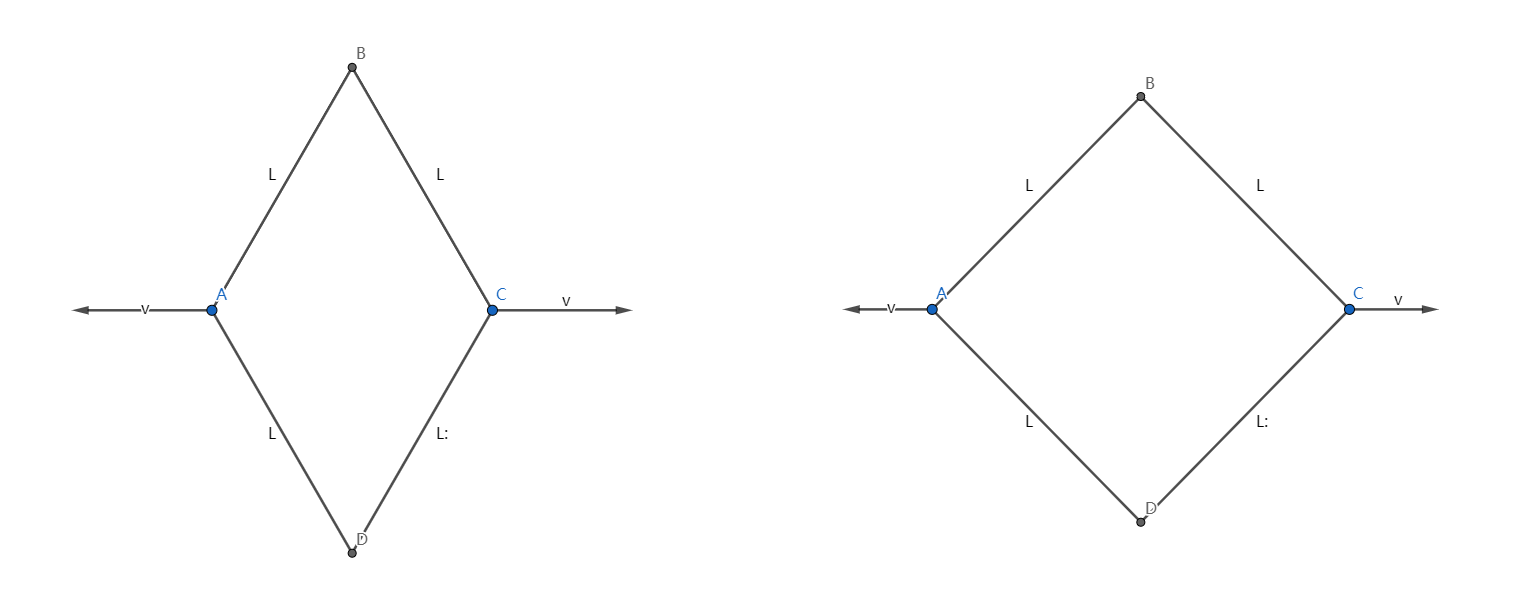# Reach for the Summit - P-S1-A5As shown above, $AB,BC,CD,AD$ are four rigid rods connected by hinges whose lengths are $L$, and it's obvious that quadrilateral $ABCD$ is a rhombus.

Initially, the diagonal $BD$ is longer than $AC$, and the rhombus is put on the horizontal ground, then $A,C$ is moving to the opposite side along line $AC$ with the same magnitude of velocity $v$.

What's the acceleration $(m/s^2)$ of point $B$ relative to the ground at the moment when the rhombus becomes a square (see the picture on the right)?

Submit the value when $L=\sqrt{2}\ m,\ v=10\ m/s$.

Reach for the Summit problem set - Physics

×